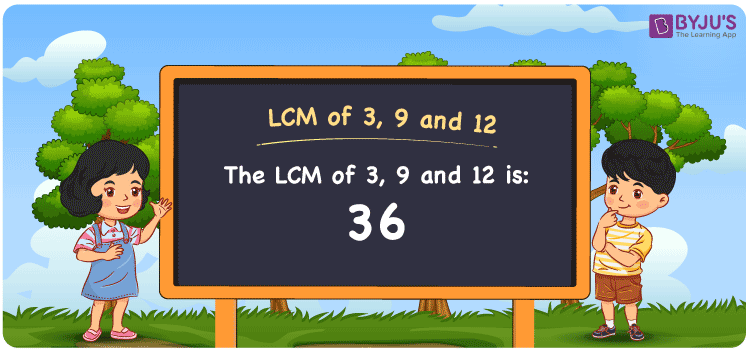# LCM of 3 9 and 12

LCM of 3, 9 and 12 is 36. LCM stands for Least Common Multiple or the Lowest Common Multiple also known as LCD Least Common Divisor is the positive number that is a common multiple to the given numbers. Here the given set of numbers are 3, 9 and 12. The smallest common multiple for all these numbers among many is 36, and hence it is the LCM. You can refer to LCM with Examples to understand better.

## What is LCM of 3, 9 and 12

The Least Common Multiple or Lowest Common Multiple of 3, 9 and 12 is 36.## How to Find LCM of 3, 9 and 12?

LCM of 3, 9 and 12 can be determined using three methods:

• Prime Factorisation
• Division method
• Listing the multiples

### LCM of 3, 9 and 12 Using Prime Factorisation Method

In the Prime Factorisation method, the numbers 3, 9 and 12 can be expressed as;

3 = 1 × 3

9 = 3 × 3

12 = 2 × 2 × 3

3 is the common factor of 3, 9 and 12. 3 along with other prime factors of 9 and 12 are multiplied to get the LCM.

LCM(3, 9 and 12) = 2 × 2 × 3 × 3 = 36

### LCM of 3, 9 and 12 Using Division Method

In the Division Method, the numbers 3, 9 and 12 are divided by common prime divisors. The product of common and other prime divisors of 3, 9 and 12 forms the LCM.

 2 3 9 12 2 3 9 6 3 3 9 3 3 1 3 1 × 1 1 1

LCM(3, 9 and 12) = 2 × 2 × 3 × 3 = 36

### LCM of 3, 9 and 12 Using Listing the Multiples

By listing all the multiples of 3, 9 and 12, we can identify the LCM. Below is the list of multiples for 3, 9 and 12

 Multiples of 3 Multiples of 9 Multiples of 12 3 9 12 6 18 24 9 27 36 12 36 48 15 45 60 18 54 72 21 63 84 24 72 96 27 81 108 30 90 120 33 99 132 36 108 144

LCM(3, 9 and 12) = 36

## Video Lesson on Applications of LCM## Solved Examples

What is the smallest number that is divisible by 3, 9 and 12?

Answer: 36 is the smallest number that is divisible by 3, 9 and 12.

What is the LCM of 3 and 36?

Answer: LCM of 3 and 36 is 36.

## Frequently Asked Questions on LCM of 3, 9 and 12

### What is the LCM of 3, 9 and 12?

The LCM of 3, 9 and 12 is 36.

### What is the difference between the LCM 3, 9 and 12 and HCF of 3, 9 and 12?

The LCM of 3, 9 and 12 is 36 and HCF of 3, 9 and 12 is 3.

### Is the LCM and LCD both the same for 3, 9 and 12?

Yes. The LCM and Lowest Common Divisor are both the same for 3, 9 and 12  which is 36.

### What are the first 3 common multiples of 3, 9 and 12?

The first three common multiples of 3, 9 and 12 are 36, 72, and 108.

### Is the LCM of 3 and 9 same as the LCM of 9 and 12?

No. LCM of 3 and 9 is 9, whereas the LCM of 9 and 12 is 36. They are not the same.